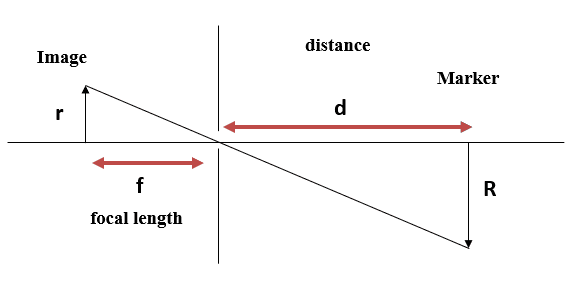## 1. Introduction

Determining the distance from an object in a video has been extensively explored in image processing and computer vision fields.

In this tutorial, we’ll demonstrate a solution to compute the distance from a camera to a known object using the most developed technique, the triangle similarity method.

## 2. Measuring Distance From the Object in Video

We can use several attempts in recent years to determine the size of an object or the distance between the camera and the object. This is because cameras are now more widely available daily since most modern mobile phones have them.

Measuring the distance between the camera and a given object is calculated through the principles of how a camera works. Calculating the distance between an object and a camera is fairly simple and straightforward if the object’s real size is known. This is due to how simple it is to comprehend how a camera operates.

The proposed method of measuring the distance to an object is among the most effective methods in computer vision presented in the literature.

We show here one specific figure of the distance between an object and a marker to understand the triangle similarity method better using the following different parameters:In order to determine the distance from a camera to a known object or marker, we’re going to utilize triangle similarity. As we can see in this figure, we have:: focal length: radius of the marker in the image plane: radius of the marker in the object plane: the unknown parameter, distance from the camera to the object

We consider the camera generates a one-to-one relationship between the object and the image. Then, we can deduce a relationship between known parameters:Subsequently, we can determine the valueas follows:## 3. Measuring Distance Example

In this part, we’ll explain a technique for measuring the distance between an object and a camera according to the operating principles of the triangle similarity method.

Let’s suppose we measure the distance from a camera to an object in a video. We know the focal length of the camerais, and the object we want to measure has a known height of. We can also see in the video that the object has a circular marker with a radius of.
Using the equation,

we can use it to solve for:Therefore, the distance from the camera to the object is approximatelyor.

## 4. Applications and Methods

Measuring distance is a technique present in various applications. In this part, we’ll speak about some examples and methods. Indeed, to set up absolute and precise distances, it is necessary to use methods providing relative distance.

For example, we need to determine the distance from a system capturing a scene in front of a car. It allows us to provide information about the detected objects and their absolute distances.

Additionally, distance information is necessary for autonomous vehicles, multi-drones, and robots. All new technologies testify to a boom in light of recent developments in Artificial Intelligence. The technology needed for the computer to detect its environment is essentially what all these new technologies require. It is one of the most crucial technologies. It allows the computer to identify and measure the distance from other nearby objects.

Currently, the radar and the lidar allow erasing to determine the distances. Radar uses electromagnetic waves that have been reflected to calculate distances. Even at night, the computer can determine distances with reasonable accuracy using radar when light is minimal. Radar readings contain errors, but these errors increase with distance from the object. Whereas, Lidar uses the reflection of its beams to measure the distance, shape, and substance of its surroundings.

### 4.1. Other Methods

Distance computation techniques have recently become an area of major research interest in the robotic and computer vision fields. So, we can find various techniques that are very uncomplicated and concise in the computer vision domain. Also, we can find complex methods using the inherent parameters of the camera model.

There are numerous methods for calculating object distance using a single fixed camera:

• The complex log mapping (CLM) method: according to the CLM methodology, the original pictures are converted from an orthogonal coordinate system to a polar one. CLM can determine the ratio between two photos since they both include properties of concentric circles
• We can find a method that allows estimating depth using a monocular camera. This method depends on the camera’s parameters and image geometry. We can use it to calculate the focal length of the camera and the camera’s field of view
• Another technique can also be used to obtain a distance measurement of an object using a single camera with a variable pitch angle improved by optimizing the least squares. This is a simple and accurate method. We can measure the distance of an object using a variable inclination angle camera. It can be defined by integrating the ratio of the angle and the pixels of a moving object

## 5. Conclusion

Generally, distance computation techniques are a very important research area in the field of computer vision and artificial intelligence. Indeed, in this tutorial, we spoke about the applications and methods to measure the distance from an object in a video. Also, we presented an efficient method to compute the distance from a camera to a known object.